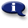# .

## Syllabus Information

Fall 2022
Dec 03,2023Use this page to maintain syllabus information, learning objectives, required materials, and technical requirements for the course.

Syllabus Information
MTH 252 - Calculus 2 (Integral Calculus)
Associated Term: Fall 2022
Learning Objectives: Upon successful completion of this course, the student will be able to:
1. Estimate & calculate totals given information about rates of change
2. Understand the definite integral as a limit of Riemann sums
3. Interpret the meaning of and use correct notation for a definite integral
4. Compute definite integrals using the first fundamental theorem of calculus
5. Understand how the definite integral and the average value of a function are related
6. Use properties and theorems pertaining to integrals
7. Graphically and numerically construct antiderivatives
8. Work with elementary differential equations
9. Work with functions defined in terms of definite integrals with a variable limit(s) of integration and apply the second fundamental theorem of calculus to the analysis of these functions
10. Understand that the indefinite integral represents a family of antiderivative functions
11. Find definite & indefinite integrals using basic rules, the substitution method, integration by parts, and trigonometric substitution
12. Use the midpoint, trapezoid, and Simpson's rule to approximate definite integrals
13. Identify improper integrals that converge or diverge and compute their values where possible
14. Use the methods & techniques of integral calculus to solve a variety of application problems
15. Use a programmable graphing calculator as an effective tool in confirming analytical work and obtaining numerical and graphical results related to integral calculus
Required Materials:
Technical Requirements: